# 【论文阅读】Faster R-CNN：Towards Real-Time Object Detection with Region Proposal Networks

## Faster R-CNN，Region Proposal Networks（RPN）

Posted by x-jeff on April 3, 2022

# 1.INTRODUCTION

EdgeBoxes原文：C. L. Zitnick and P. Doll´ar, “Edge boxes: Locating object proposals from edges,” in European Conference on Computer Vision (ECCV), 2014.。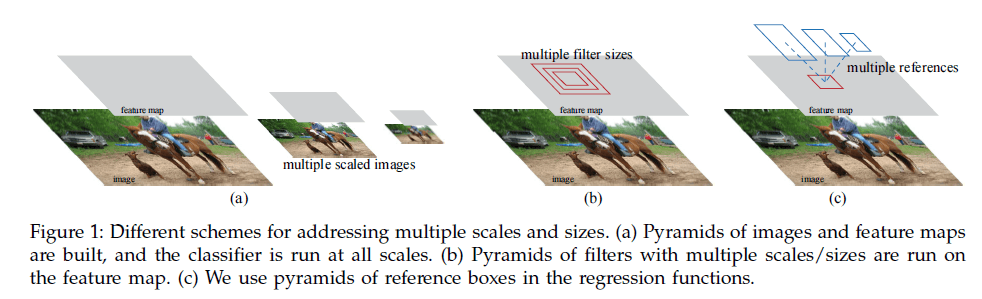# 3.FASTER R-CNN

Faster R-CNN包含两个模块。第一个模块是用于生成proposal的深度全卷积网络，第二个模块是使用这些proposal的Fast R-CNN检测模型。如Fig2所示，Faster R-CNN是一个单一的模型（即不再需要把proposal的生成和检测任务分割开来）：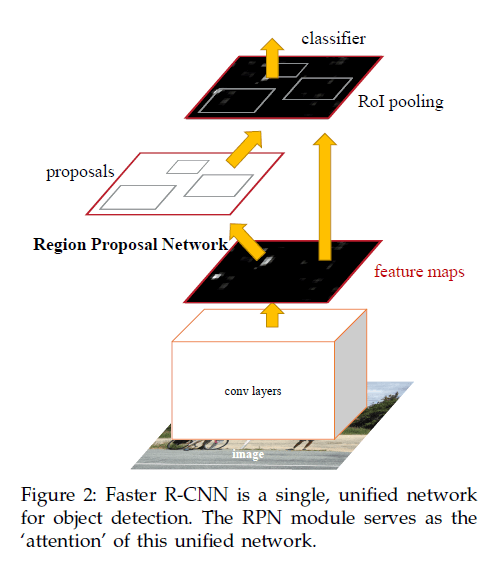## 3.1.Region Proposal Networks

RPN的输入为任意大小的图像（或feature map），输出为一系列矩形object proposal，并且每个proposal都有一个评分（用于评价属于前景还是背景）。本文中，我们使用FCN作为RPN。因为我们最终目的是让RPN和Fast R-CNN目标检测网络可以共享计算，因此我们让其共享卷积层，如果是基于ZFNet，则可以共享5个卷积层；如果是基于VGG16，则可以共享13个卷积层。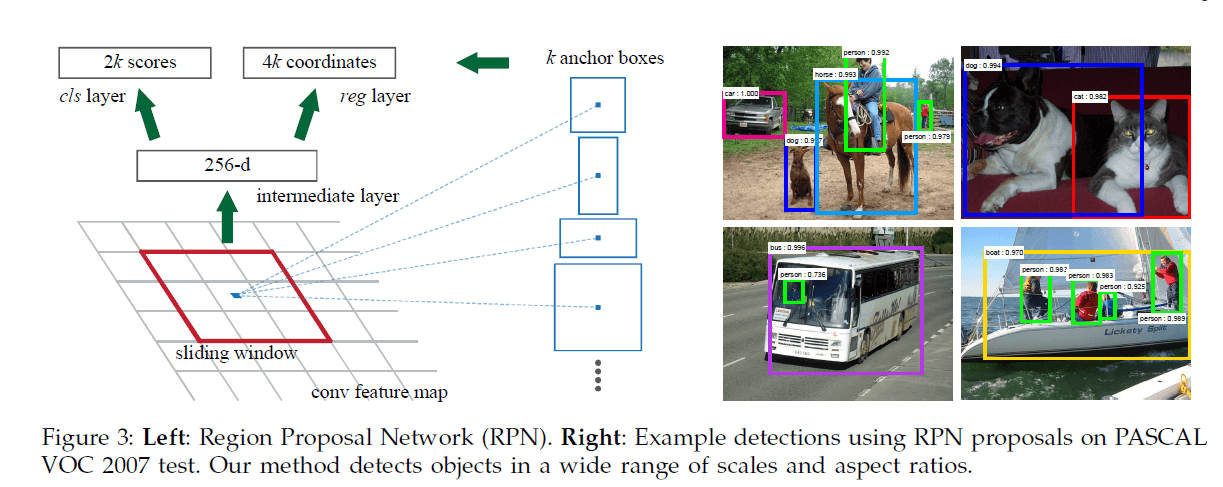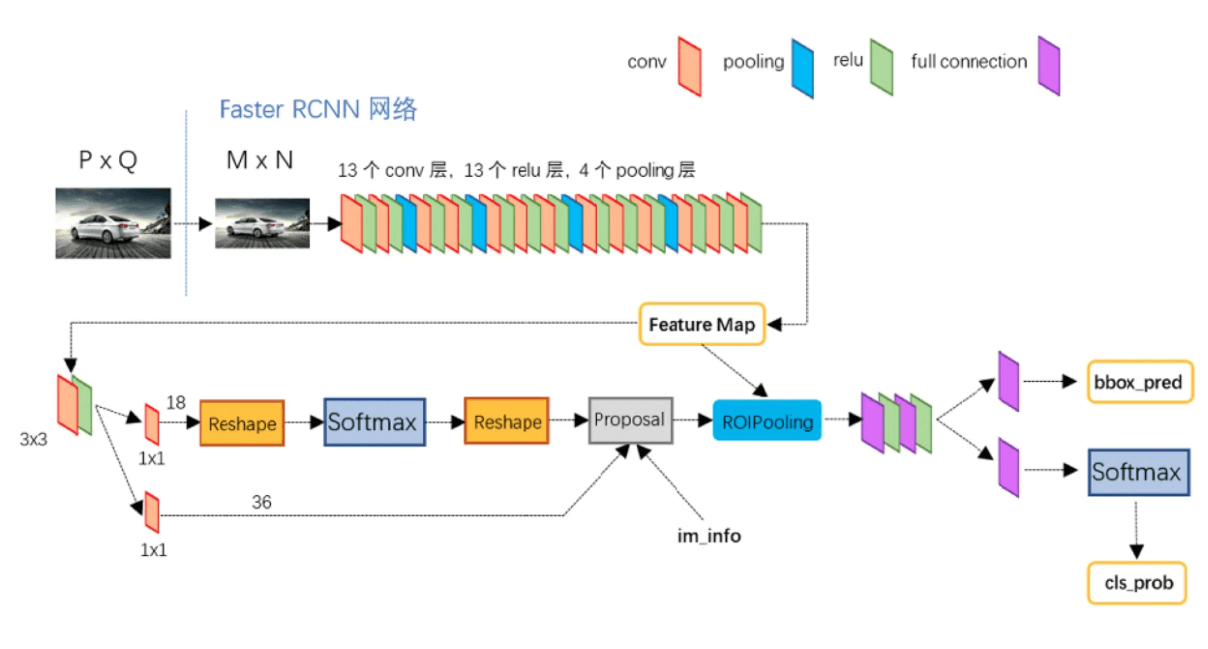### 3.1.1.Anchors

👉Translation-Invariant Anchors

anchor机制具有平移不变性。平移不变性使得我们模型的size更小（即更少的参数数量），这同时也降低了过拟合的风险。

👉Multi-Scale Anchors as Regression References

### 3.1.2.Loss Function

$L( \{ p_i \},\{ t_i \})=\frac{1}{N_{cls}} \sum_i L_{cls} (p_i,p^*_i)+\lambda \frac{1}{N_{reg}} \sum_i p^*_i L_{reg} (t_i,t^*_i) \tag{1}$

$i$表示在一个mini-batch中第$i$个anchor的索引，$p_i$为第$i$个anchor属于前景的概率。$p_i^*$是GT，当第$i$个anchor为正样本时，$p_i^*=1$；为负样本时，$p_i^*=0$。$t_i$（预测值）和$t_i^*$（GT）为四个值的向量，定义和R-CNN一致：

$\begin{split} t_x = (x-x_a)/w_a, \quad t_y=(y-y_a)/h_a, \\ t_w=\log(w/w_a), \quad t_h=\log(h/h_a), \\ t^*_x=(x^*-x_a)/w_a, \quad t^*_y=(y^*-y_a)/h_a, \\ t^*_w=\log(w^*/w_a), \quad t^*_h=\log(h^*/h_a), \end{split} \tag{2}$

SPPnetFast R-CNN中，所有region proposal在经过spatial pyramid pooling layer后的bounding box回归计算使用的同一组权重。而我们的方法针对每一种类型的anchor都训练了一个单独的回归器，即共训练了$k$个不同的回归器，每个回归器只负责一种scale和aspect ratio的anchor。

## 3.2.Sharing Features for RPN and Fast R-CNN

👉（i）Alternating training.

👉（ii）Approximate joint training.

👉（iii）Non-approximate joint training.

Faster R-CNN并没有使用这种方法，不再赘述。

👉4-Step Alternating Training.

1. 按照第3.1.3部分训练RPN。使用ImageNet-pre-trained的模型来初始化RPN。
2. 使用第1步生成的proposal，开始单独训练Fast R-CNN。同样的，也使用ImageNet-pre-trained的模型来初始化Fast R-CNN。此时，RPN和Fast R-CNN还未开始共享卷积层。
3. 使用Fast R-CNN初始化RPN，然后固定需要共享的卷积层，fine-tune只属于RPN的层。此时，RPN和Fast R-CNN就开始了共享卷积层。
4. 最后，依旧固定共享的卷积层，fine-tune只属于Fast R-CNN的层。

## 3.3.Implementation DetailsRPN产生的一些proposal重合度很高。我们根据cls分数进行NMS，IoU的阈值设为0.7，这样的话每幅图像差不多能剩2000个左右的proposal。NMS不但大量减少了proposal的数量，并且还没有损害最终的检测准确率。在NMS之后，我们选择排名前$N$的proposal进行检测（个人理解：按照cls分数进行排名）。在训练阶段，我们使用2000个由RPN生成的proposal来训练Fast R-CNN，在测试阶段，我们评估了使用不同数量的proposal。

# 4.EXPERIMENTS

## 4.1.Experiments on PASCAL VOC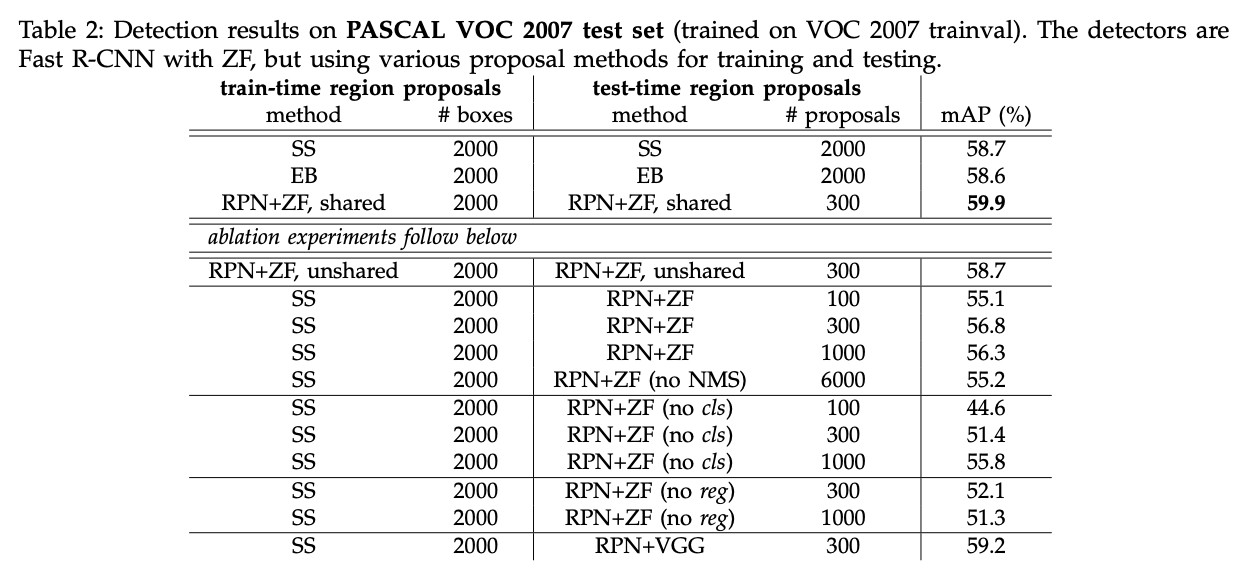👉Ablation Experiments on RPN.

SS+300个RPN proposal得到了最高的mAP，为56.8%。mAP的降低是因为训练和测试阶段的proposal方法不一样。该结果作为以下比较的baseline。

👉Performance of VGG-16.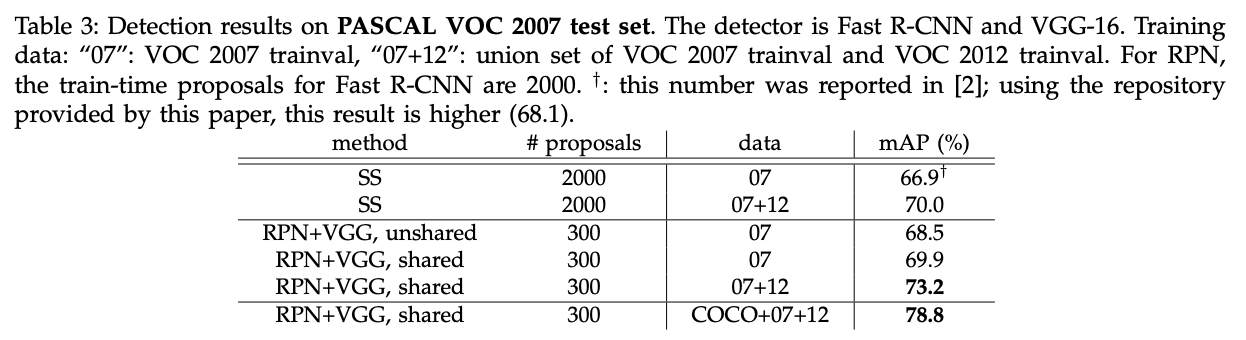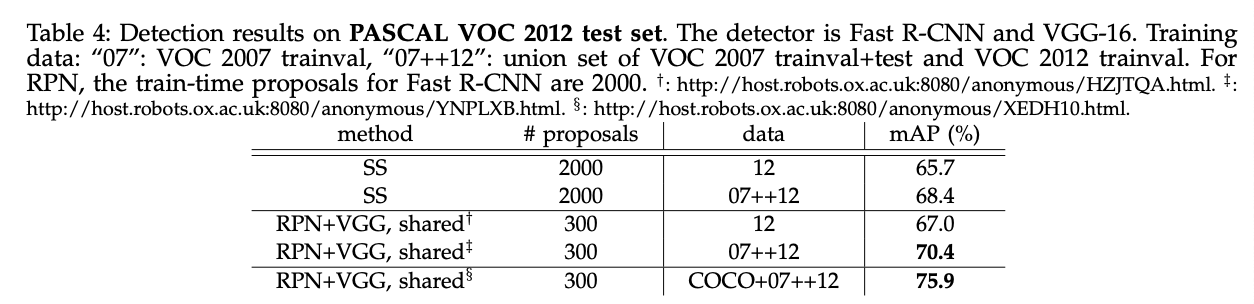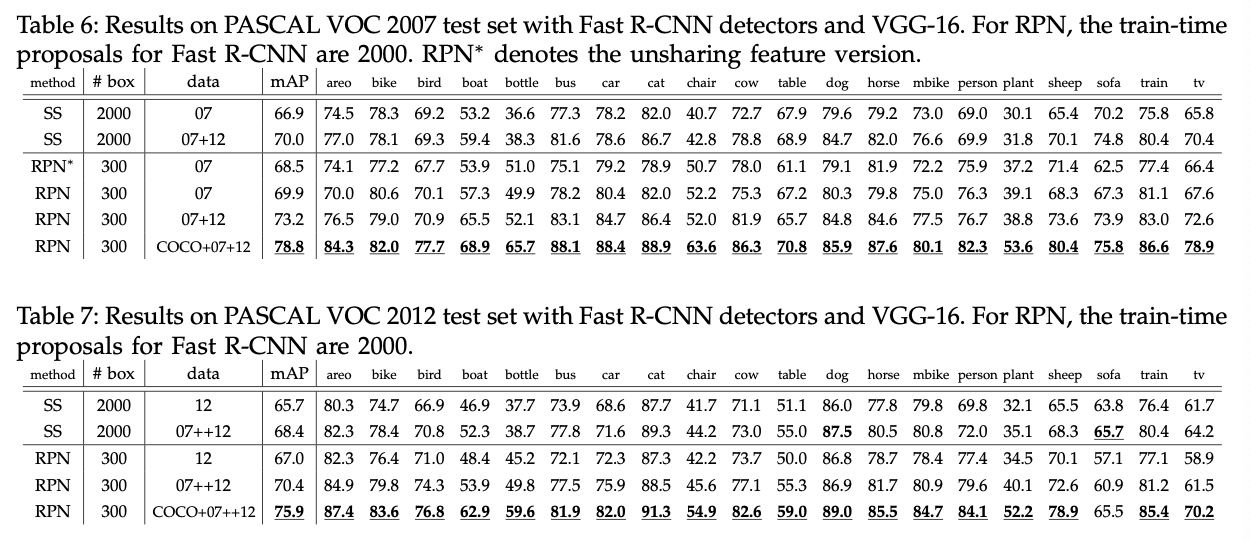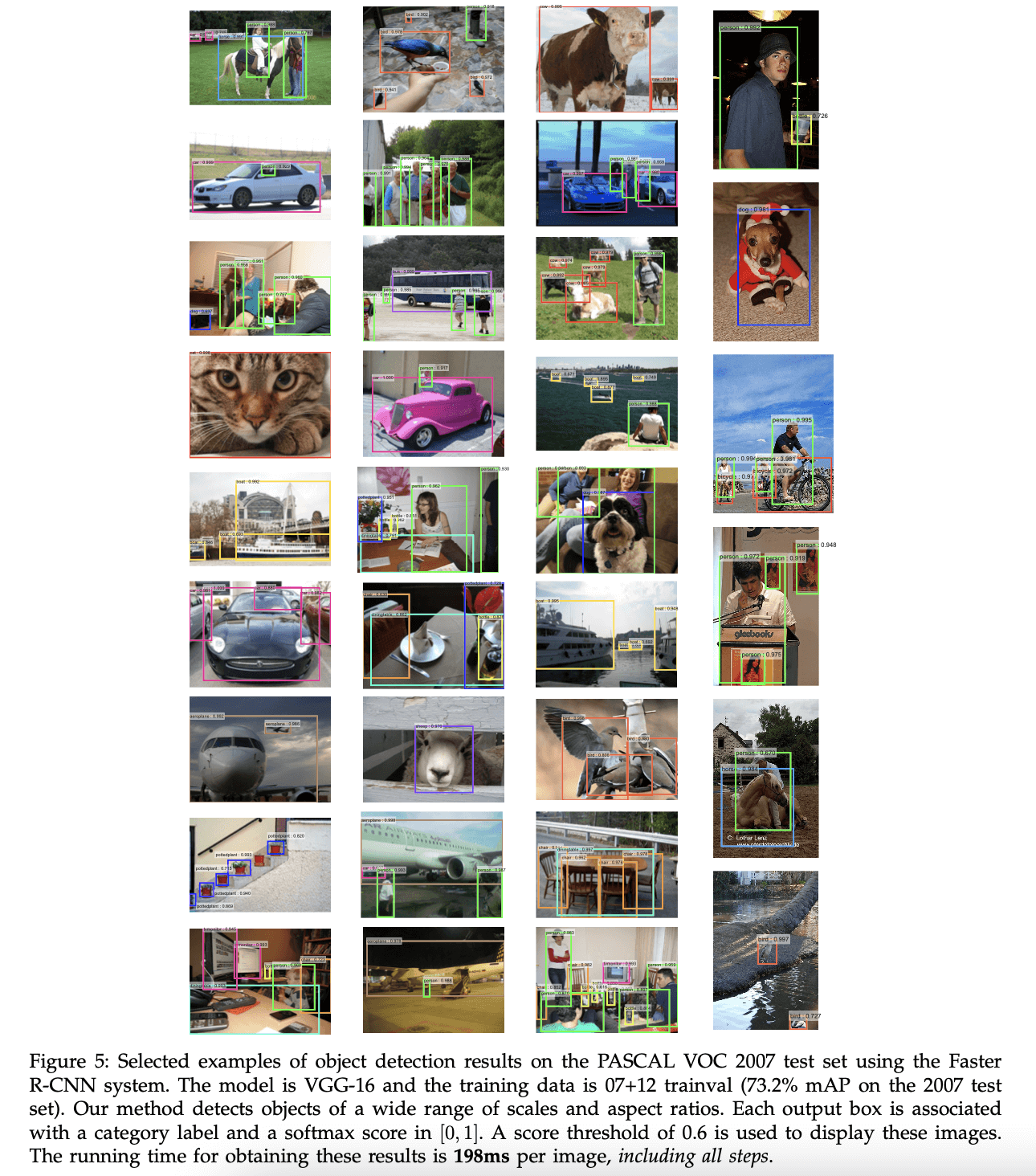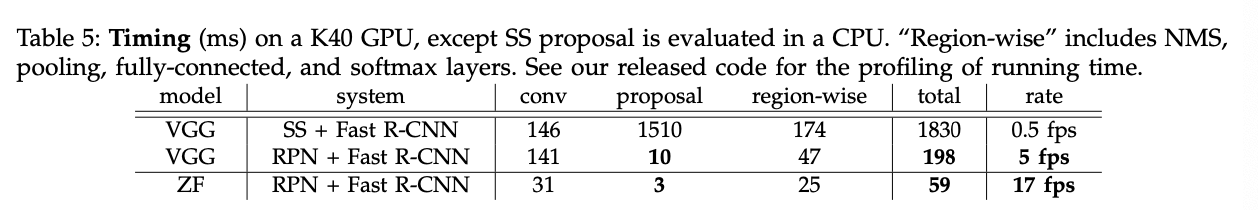👉Sensitivities to Hyper-parameters.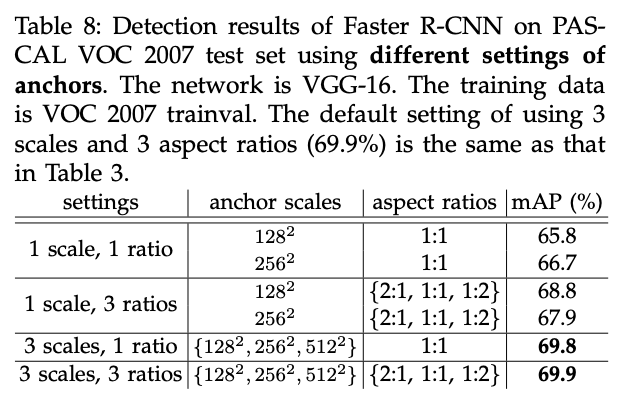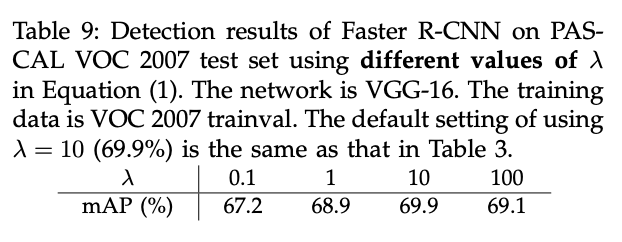👉Analysis of Recall-to-IoU.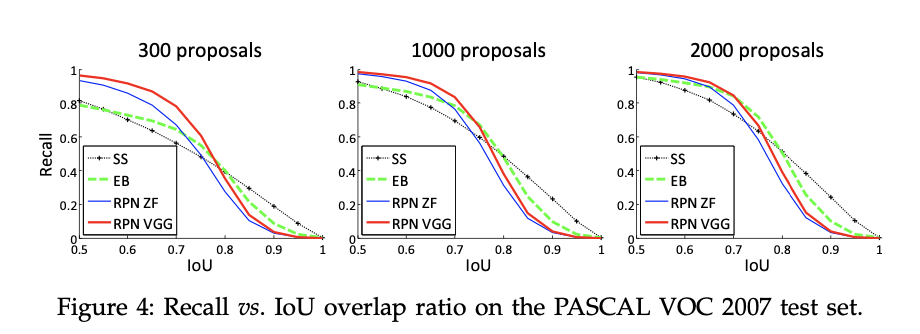👉One-Stage Detection vs. Two-Stage Proposal + Detection.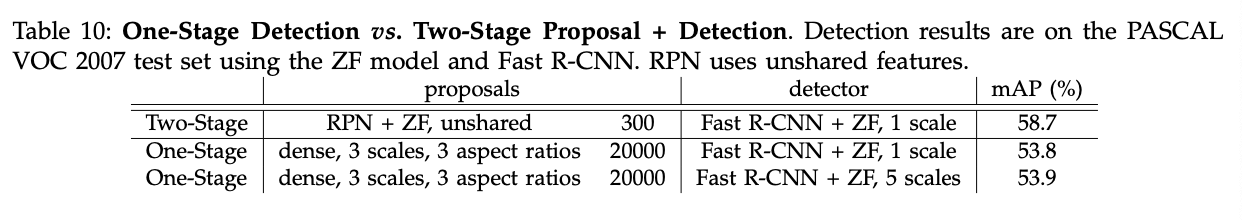## 4.2.Experiments on MS COCO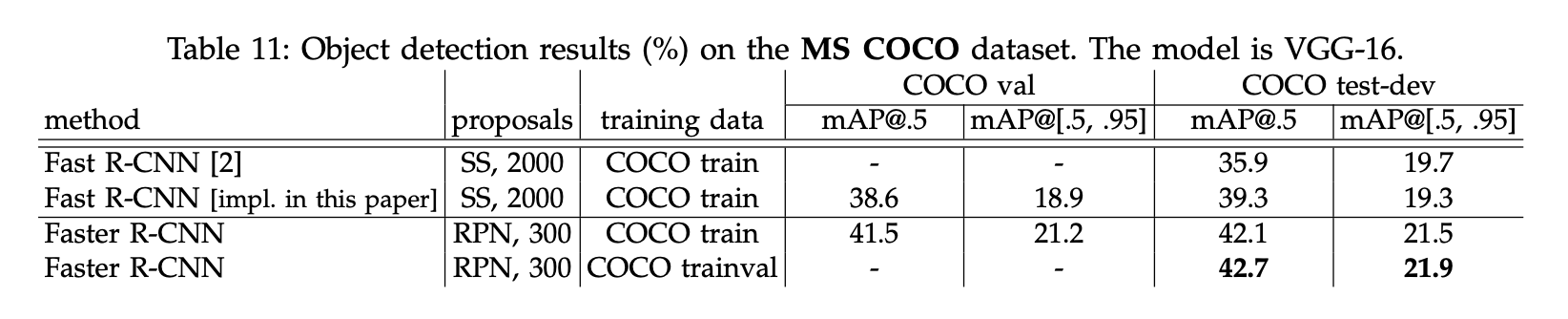👉Faster R-CNN in ILSVRC & COCO 2015 competitions

## 4.3.From MS COCO to PASCAL VOC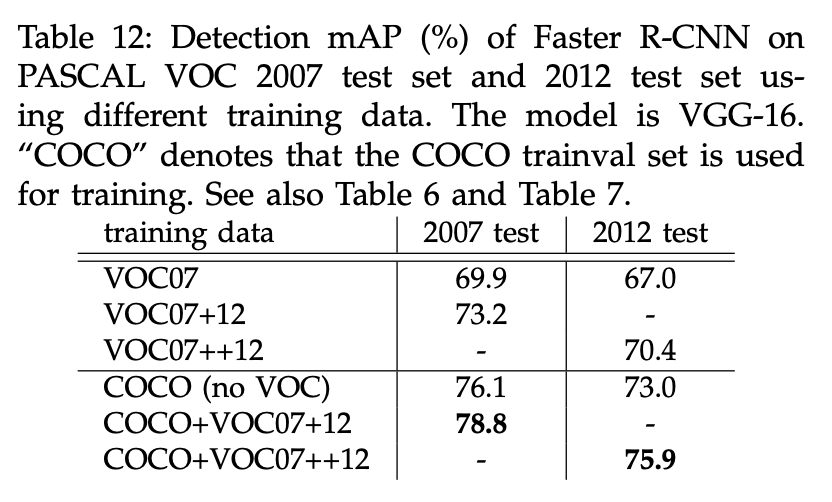# 6.原文链接